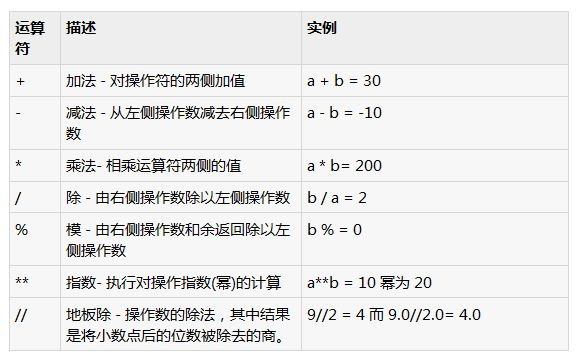# 举例讲解Python中的算数运算符的用法#!/usr/bin/python

a = 21
b = 10
c = 0

c = a + b
print "Line 1 - Value of c is ", c

c = a - b
print "Line 2 - Value of c is ", c

c = a * b
print "Line 3 - Value of c is ", c

c = a / b
print "Line 4 - Value of c is ", c

c = a % b
print "Line 5 - Value of c is ", c

a = 2
b = 3
c = a**b
print "Line 6 - Value of c is ", c

a = 10
b = 5
c = a//b
print "Line 7 - Value of c is ", c

Line 1 - Value of c is 31
Line 2 - Value of c is 11
Line 3 - Value of c is 210
Line 4 - Value of c is 2
Line 5 - Value of c is 1
Line 6 - Value of c is 8
Line 7 - Value of c is 2

Tools Online | 在线开发工具
RankList | 热门文章Recommend | 推荐阅读
PHP1.CN | 中国最专业的PHP中文社区 | PHP资讯 | PHP教程 | 数据库技术 | 服务器技术 | 前端开发技术 | PHP框架 | 开发工具 | PHP问答